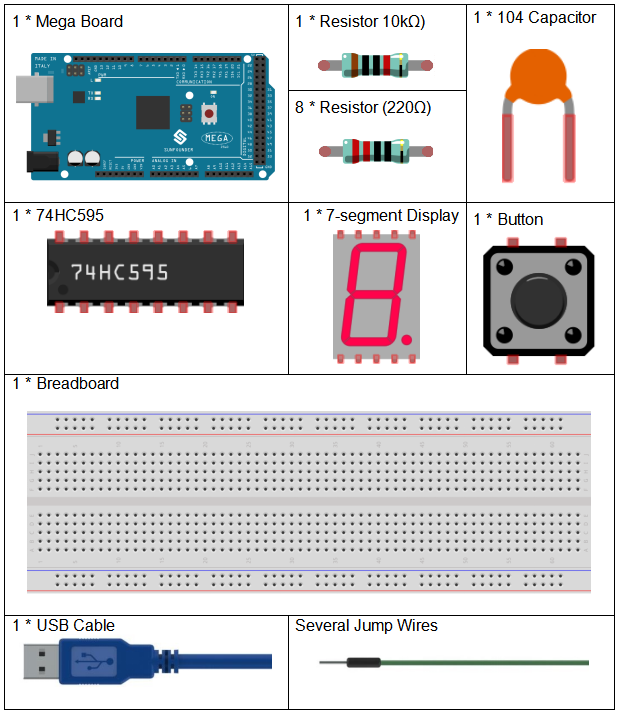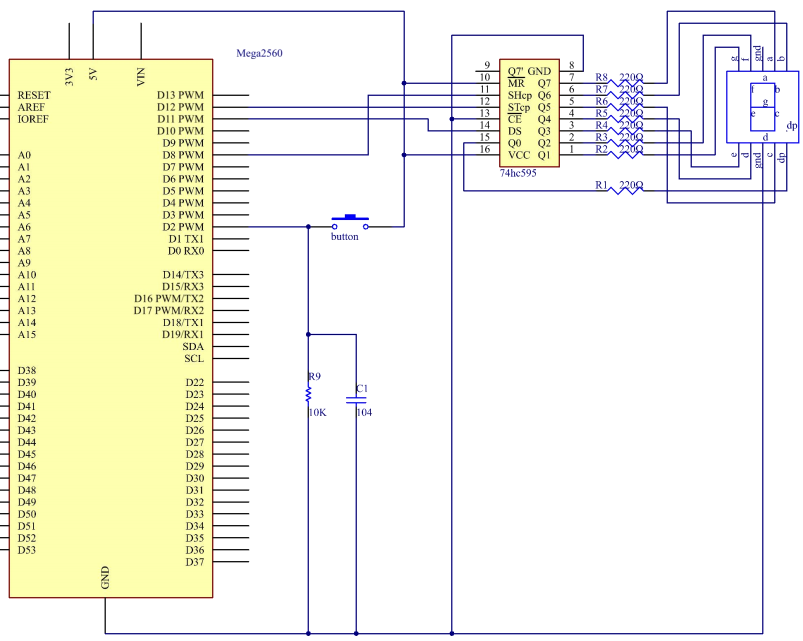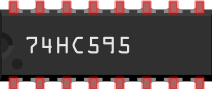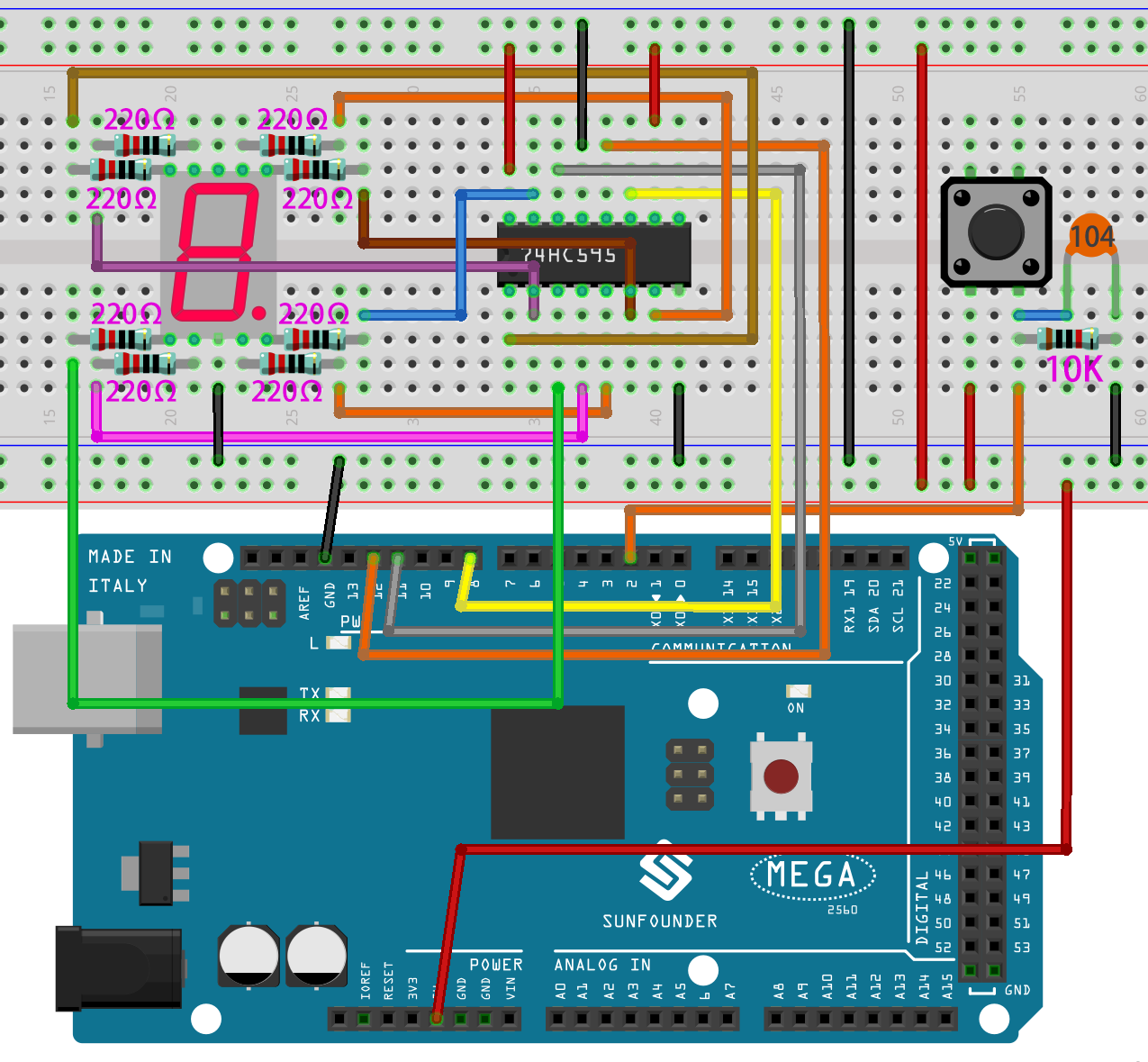# Lesson 23 Simple Creation - Digital Dice¶

## Introduction¶

In previous experiments, we learned how to use a 7-segment display and control LEDs by a button. In this lesson, we will use a 7-segment display and a button together to create a simple digital dice.

## Components¶## Schematic Diagram¶

The idea behind a digital dice is very simple: a 7-segment display circularly jumps from 1 to 7 rapidly. When the button is pressed, the jumping will slow down until it stops on a number. When the button is pressed again, the process will repeat.

The schematic diagram:## Experimental Procedures¶

Step 1: Build the circuitStep 2: Open the code file.

Step 3: Select the Board and Port.

Step 4: Upload the sketch to the board.

You can now see the 7-segment display jump between numbers from 1 to 6. Press the button, and the jumping will slow down until it stops three seconds later. Press the button again, and the process will repeat.## Code Analysis¶

The initial random number comes from A0

```randomSeed(analogRead(0));
```

The initial random number is generated from A0 and the range for the random numbers is 0-1023.

Digital Dice

```void loop()
{
if(stat == HIGH)  // check if the pushbutton is pressed
```

If yes, the corresponding pin is high level.

```{
num ++; // num adds 1
if(num > 1)
{
num = 0;
}
}
```

If num > 1, clear the value. This is to prevent repeated pressing. So just count it as once no matter how many times you press.

```Serial.println(num);  // print the num on serial monitor
if(num == 1)  //when pushbutton is pressed
{
randNumber = random(1,7); //Generate a random number in 1-7
showNum(randNumber);  //show the randNumber on 7-segment
delay(1000);  //wait for 1 second
while(!digitalRead(keyIn));  //When not press button,program stop here.
```

Make it keep displaying the last random number.

```int stat = digitalRead(keyIn);
```

Read the state of the button again.

```if(stat == HIGH) // check if the pushbutton is pressed
```

If yes, run the code below.

```      {
num ++; // num+1=2
digitalWrite(ledPin,HIGH); //turn on the led
delay(100);
digitalWrite(ledPin,LOW); //turn off the led
delay(100);
if(num >= 1) // clear the num
{
num = 0;
}
}
}
//show random numbers at 100 microseconds intervals
//If the button has not been pressed
randNumber = random(1,7);
showNum(randNumber);
delay(100);
}
```

showNum() function

```void showNum(int num)

{

digitalWrite(latchPin,LOW); //ground latchPin and hold low for transmitting

shiftOut(dataPin,clockPin,MSBFIRST,datArray[num]);

//return the latch pin high to signal chip that it

//no longer needs to listen for information

digitalWrite(latchPin,HIGH); //pull the latchPin to save the data

}
```

This function is to display the number in `dataArray[]` on the 7-segment display.